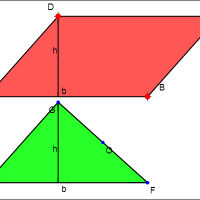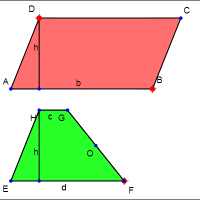## Common Core Nuggets :: Area Formulas

This collection contains apps that will help teachers implement Common Core Standard 6.G.1 for 6th grade mathematics.#### Area of a Parallelogram

This app allows you to compare areas of a parallelogram and a rectangle in order to discover the formula for the area of the parallelogram.#### Area of a Triangle

This app allows you to compare areas of an arbitrary triangle and a parallelogram in order to discover the formula for the area of the triangle.#### Area of a Trapezoid

This app allows you to compare areas of an arbitrary trapezoid and a parallelogram in order to discover the formula for the area of the trapezoid.